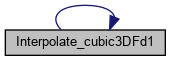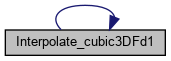FLASH-X Doxygen Generated Documentation From Interface Source Code
Interpolate_cubic3DFd1.F90 File Reference

Go to the source code of this file.

## Functions/Subroutines

real function, dimension(1:4) Interpolate_cubic3DFd1 (a, x, y, z)

## ◆ Interpolate_cubic3DFd1()

 real function, dimension (1:4) Interpolate_cubic3DFd1 ( real, dimension (1:64), intent(in) a, real, intent(in) x, real, intent(in) y, real, intent(in) z )

Calculates the function value and all rescaled 1st derivative values for a triple [x,y,z] of rescaled [0,1] coordinates and the 64 tricubic expansion coefficients. The tricubic expansion reads, for one cube, in terms of rescaled [0,1] x,y,z coordinates:

3 3 3 i j k F (x,y,z) = sum sum sum a (i,j,k) x y z i=0 j=0 k=0

The order of the supplied expansion coefficients a (i,j,k) must be such, that the k index has the highest ranking, followed by the j index and the i index. The overall location index of the a (i,j,k) inside the 64-dimensional vector is given by the following formula:

location index of (i,j,k) = 1 + i + 4j + 16k

The rescaled derivatives are given by the general formula:

r+s+t r s t 3 3 3 i-r j-s k-t d / dx dy dz = sum sum sum (i) * (j) * (k) * a (i,j,k) * x y z i=r j=s k=t r s t

where the Pochhammer symbols are defined as:

(i) = i * (i-1) * (i-2) * ... * (i-r+1) r

The rescaled derivatives are therefore also sums of appropriate expansion coefficients times monomial products. From the general formula we see, that the highest non-zero derivative is of 9-th order.

Since this function is (potentially) called many times from external applications, efficiency is key here and intermediate common summation terms are reused as much as possible. The strategy is partial summation and reduction at each index summation stage. The individual x-,y- and z-coordinate cubic polynomial sections are always evaluated using the Horner scheme to minimize accumulation of computation rounding errors.

a (i) : the i-th tricubic expansion coefficient x : rescaled [0,1] x coordinate y : rescaled [0,1] y coordinate z : rescaled [0,1] z coordinate

NOTES

1) The function is defined as a real array of size 4:

Interpolate_cubic3DFd1 (1) = the function value Interpolate_cubic3DFd1 (2) = the rescaled d/dx value Interpolate_cubic3DFd1 (3) = the rescaled d/dy value Interpolate_cubic3DFd1 (4) = the rescaled d/dz value

2) The code checks, if the supplied triple [x,y,z] is rescaled.

Definition at line 72 of file Interpolate_cubic3DFd1.F90.

References Interpolate_cubic3DFd1().

Referenced by Interpolate_cubic3DFd1().

Here is the call graph for this function:Here is the caller graph for this function: